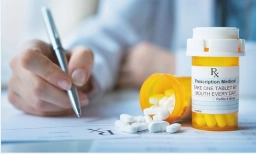# Effectiveness 80811

According to clinical studies, the effectiveness of the drug is 90%. The doctor prescribed the medicine to eight patients. What is the probability that the drug will be effective in all these patients?

p =  0.4305

### Step-by-step explanation:Did you find an error or inaccuracy? Feel free to write us. Thank you!

Showing 1 comment:
Math student
The effectiveness of the drug is 90% or 0.9, which means that the probability of the drug being effective for any given patient is p = 0.9. We can use the binomial distribution to calculate the probability of the drug being effective in all eight patients, assuming that each patient is independent.

Let X be the number of patients for whom the drug is effective. The number of trials is n = 8, and the probability of success is p = 0.9. The probability of getting exactly k successes out of n trials is given by the binomial distribution formula:

P(X = k) = (n choose k) * p^k * (1-p)^(n-k)

where (n choose k) is the number of ways to choose k successes out of n trials, which is given by the binomial coefficient.

To find the probability of the drug being effective in all eight patients, we need to calculate the probability of getting exactly eight successes:

P(X = 8) = (8 choose 8) * 0.98 * (1-0.9)^(8-8)
= 0.98
= 0.43046721

Therefore, the probability of the drug being effective in all eight patients is approximately 0.4305 or 43.05%. This means that there is a 43.05% chance that all eight patients will respond to the drug and have positive outcomes.

Tips for related online calculators
Looking for a statistical calculator?